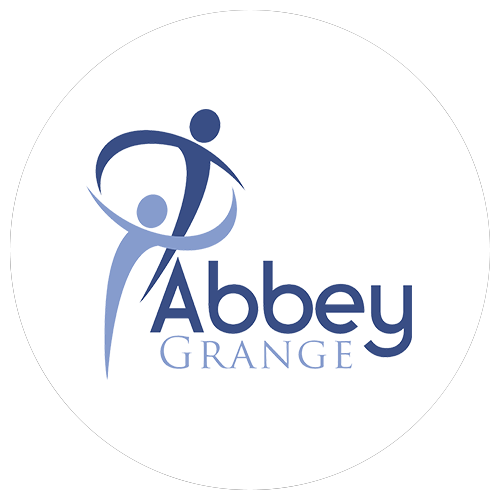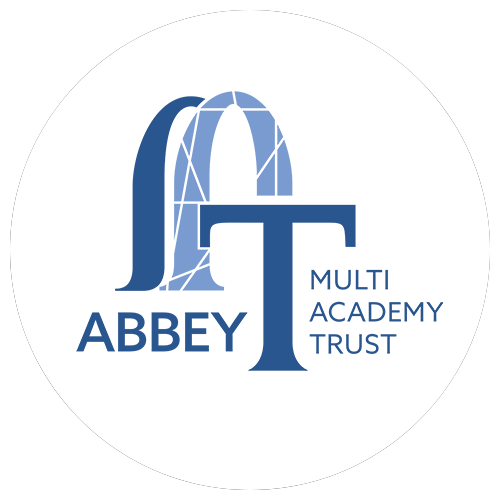# Develop Mathematical thinking

• Using a variety of concepts and models to develop mathematical explanations and understanding.
• Explaining every day and technological applications of mathematics.
• Use probability and statistical methods to evaluate valuating risks both in a practical world and the wider societal context.
• Introduce new and enhance existing prior learning from KS2.

## Develop problem solving and reasoning

• To enable pupils’ thinking and articulating their reasons in problem-solving context.
• To enable increased confidence in students to tackle unfamiliar and non-routine problems, some of which may be multi-step.
• Considering many ideas for developing problem solving tasks in standard topics across KS3
• Adapting standard questions to make teaching richer and more meaningful.

## Promote an appreciation of the links between different elements of Mathematics

• Maths fluency is knowing the key mathematical facts and methods that run through all topics within the subject and being able to recall them efficiently.
• Linking fractions, decimals and percentages.
• Using algebra to solve geometrical problems.
• Using ratio in a myriad of applications to ensure and early understanding.

## Use Mathematics to better explain the world around us

• Maths is everywhere, from calculating your holiday money using currency conversions, to calculating the interest on your savings account.
• How the use of algebra can be used to solve many real life problems such as calculating unknown costs and distances.
• How the price of sale items and discount offers can be compared in supermarkets to purchase best value items.
• Knowing ratio and proportion to exchange money, calculate ingredients for recipes.
• Using an appropriate number of significant figures in calculations to estimate values.

# Useful Resources

There are no further resources for this subject.

At Abbey Grange Academy the Key Stage 4 Maths Curriculum:

• It is the aim of the Maths department to educate students with the foundations of essential mathematical knowledge they need for life
• To nurture an appreciation for the beauty and history of Mathematics and the contribution it has made, and continues to make to the ever changing world around us.
• To empower all of our students to become problem solvers and develop a resilience that will not only stand them in good stead for future learning, but will remain with them for life.

# In Maths, we aim to:

## Develop Mathematical thinking

• the ways in which mathematical methods and theories develop over time.
• using a variety of concepts and models to develop mathematical explanations and understanding.
• appreciating the power and limitations of maths and considering ethical issues which may arise.
• explaining every day and technological applications of maths; evaluating associated personal, social, economic and environmental implications; and making decisions based on the evaluation of evidence and arguments.
• recognising the importance of peer review of results and of communication of results to a range of audiences.

## Develop problem solving and reasoning

• using mathematical theories and explanations to develop hypotheses.
• applying a knowledge of a range of techniques to analyse and solve problems.
• understanding the impact of the accuracy of measurements.

## Promote an appreciation of the links between different elements of Mathematics

• collecting, presenting and analysing data.
• using algebra within geometry to find missing lengths and areas.
• translating data from one form to another.
• identify patterns and trends in sequences and linking these to algebraic equations and graphs.
• use of fractions as a means of representing proportion and probability
• presenting reasoned explanations.
• use of practical maths involving percentage change and developing into growth and decay. This links between financial and biological aspects of maths.

## Use Mathematics to better explain the world around us

• understanding the aspects of financial maths in our daily lives.
• use of algebra as a tool for solving problems around us such as modelling trajectories.
• model mathematical situations and express the results using formal mathematical representations.
• use of formula to find areas and volumes of everyday shapes, and using these to evaluate mass density and volume problems.
• overcoming architectural problems using trigonometry and constructions.
• knowing ratio and proportion to exchange money, calculate ingredients for recipes and best values for money.
• Using an appropriate number of significant figures in calculations to estimate values.

# Useful Resources

• Corbett maths
• Hegarty maths,
• Maths genie
• Brilliant.org

# Abbey Grange C of E Academy

Butcher Hill
Leeds
LS16 5EA

Tel: 0113 275 7877
Fax: 0113 275 4784

# Registered Office:

c/o Chapter House
Abbey Grange Church of England Academy
Butcher Hill
Leeds
LS16 5EA
Registered Company Number: 07705552